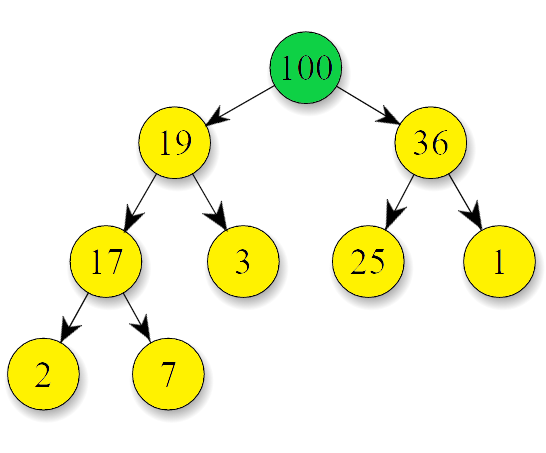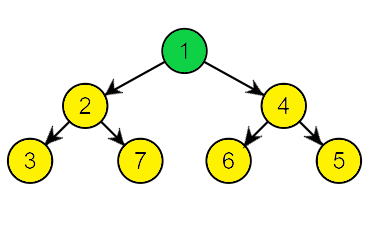# Max Heap vs Min Heap

In this article, we will have a look at the difference between Max Heap and Min Heap Data Structure. We will look at the description of each data structure and examples related to them.

Firstly, let us have a quick recap of the Heap Data Structure. Heap is a special type of binary tree based structure. The underlying structure resembles that of a Complete Binary Tree. As, it is a tree-based data structure all the nodes of the tree are arranged in a specific order. The tree arranges it’s nodes on the basis of a priority. Hence, it is useful to remove the highest or lowest priority element depending on the type of the heap. Typically, we represent heap using an array for faster access and space efficiency. There are mainly two types of heap: Max Heap and Min Heap. Let us have a look at the two types.

### Max Heap

A Max Heap is basically a complete binary tree in which the Key/Data present at the Root node is greater than or equal to the data present at all of its child nodes. In other words, the Root node has the Maximum value. This property must be true for every sub-tree. So, for every Internal Node or for every pair of parent and it’s child node in the heap, the node has always a greater value than it’s descended child nodes. Internal Node is a node that has at least one children (At Most two). The height of a Max Heap of N nodes is log(N).

Let us look at the example of Max Heap:Above a max heap is shown, The Root node (100) is highlighted to show the Max Heap property. We can see it is the node with the maximum value. Along with this, the Key/Data of each Parent Node is greater than it’s corresponding child node As, we traverse down each level (starting from the root) the value of nodes decrease.

#### Operations On Max Heap with Time Complexities

• Get Maximum: This is used to get the maximum element from the heap which in turn just returns the Root element of the Heap . The Time Complexity is O(1) for this operation.
• Extract Maximum: This operation removes the root node with maximum value from the tree and return it. After removing the root we have to again balance the tree to maintain the heap property. The Overall Complexity is O(log n).
• Insert: To insert a new node we add the node at the end of tree, while ensuring the heap property stands true otherwise we balance the tree. The Complexity is also O(log n).

### Min Heap

A Min Heap just like a Max Heap follows the same structural representation, the differences arise in the ordering of the nodes. The Key/Data present at the Root node is less than or equal to the data present at all of it’s child nodes. Here, The Root has the Minimum value among all nodes. Again, this property must be recursively true for each of the subtree i.e. for every pair of parent and child node, the node value of parent node must be smaller than it’s child nodes.

Below a typical Min-Heap is shown:As we can see the Root Node (1) is the smallest among all nodes in the heap. The value of each parent node is smaller than it’s child nodes. As we traverse down from the root to the leaves the value decrease at each level.

#### Operation on Min Heap with Time Complexities

• Get Minimum: This operation gets the minimum element from the heap which in turn just returns the Root element of the Heap. The Time Complexity is O(1).
• Extract Minimum: This operation removes the root node having the minimum value in the heap and return it. After removing the root we have to again balance the tree to maintain the heap property. The Overall Complexity is O(log n).
• Insert: In order to insert a new node we add the node at the end of tree, while ensuring the heap property stands true otherwise we balance the tree. The Complexity here is also O(log n).

Now, we will look at some key differences between Max Heap and Min Heap.

## Difference between Max Heap and Min Heap

 Max Heap Min Heap 1. In Max Heap the data present at the root node must be greater than or equal to data of all it’s child nodes. 1. In Min Heap the data present at the root node must be less than or equal to data of all other nodes. 2. The Root node has the maximum value. 2. The Root node has the minimum value. 3. In Max Heap the node with largest value has the highest priority. 3. In Min Heap the node with smallest data has the highest priority. 4. In Max Heap, we extract the maximum value first. 4. In Min Heap, we extract the minimum value first. 5. The operations performed in Max Heap are: Get Maximum Element, Extract Maximum and Insert. 5. The operations performed in Min Heap are: Get Minimum, Extract Minimum and Insert. 6. Max Heap is used in Heap Sort to sort elements in ascending order. It is also used to implement a Priority Queue. 6. Min Heap is used in Heap Sort if we want to sort the data in descending order. It is also used in implementing Dijkstra’s Graph Algorithm.

That’s it for the article, we had a look at the key differences between Max Heap and Min Heap with some examples.

You can leave your suggestions or questions in the comment section below.